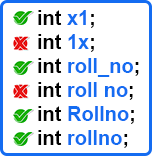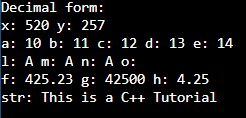# Variables and Literals in C++

## Variables and Literals in C++ with Examples:

In this article, I am going to discuss Variables and Literals in C++ with Examples. Please read our previous article where we discussed the Size and Range of Data Types in C++ with Examples.

##### Variables in C++:

Variables are useful for storing the data inside a program. During the runtime of a program, the program will store the data in variables. Variables are also called identifiers or a name given by any programmer. Variables must be declared before their use. So, when we declare a variable then it will occupy some memory during the execution time of a program. Let us see how to declare and use a variable. Suppose we want to store the marks of some subjects, so we will write,

int marks;

This statement is called the declaration of a variable. Here int is the data type and marks is the variable name. You can mention the data type based on the type of data you want to store in a variable. Marks are integers, so we have taken the int data type. Now next thing is if you want to assign some initial value to a variable so you can directly assign it.

int marks = 20;

This is known as initialization. Initialization is the first value given to a variable when it is declared. The direct value assigned to a variable is called literal. Here 20 is literal. Now for a variable, the very first thing is how we should give a variable name. Let us learn about this.

##### Variable Naming Rules in C++:
2. Variable names can contain alphabet or numbers.
3. No special characters are allowed except underscore (_).
4. C++ is a case-sensitive language. So ‘Float’ is different from ‘float’.

We can declare a variable x1,

int x1;

but we cannot declare a variable 1x. We can declare roll_no but not ‘roll no’. This is not allowed. ‘Roll_no’ and ‘roll_no’ are two different variables.You can see the correct variable naming in the above image. It is a good practice to give only meaningful variable names. So, we can write,

int rollno;
int roll_no;
int RollNo;

We can declare any of them. Out of these three, ‘RollNo’ and ‘roll_no’ are more readable. We can use underscore or capital letters to separate the words in the variable name. Now let us look at the declaration. When we declare a variable, it should have its data type. And if we are declaring multiple variables then we can write,

int x1, x2, x3;

These are three variables that we have declared at once only. If you want to store decimal point numbers then we can use float data type as,

float y1, y2, y3;

So, we can declare multiple variables in a single line for any data type. So multiple declarations are allowed. Now next point is selecting the data type. It depends on you. The type of data you want to store in a variable, so depending on this you can select the data type. If the number is larger than the integer range then you can use long data type or else you can use long long also. And if the decimal point number is larger, you can use double or long double also. That all depends on the data. Now let us see how we can initialize a value to a variable.

Suppose we want to store the day of the week. We want to store 1st day so,

int day = 1;

We have declared and initialized the day with 1. This is one method of assigning. We can do the same thing as,

int day (1);
int day = (1);
int day {0};
int day = {0};

These are the 5 methods by which we can assign a value. Commonly programmers use the first one. But we also have these methods available in C++. These methods were introduced in C++ version 11. Earlier there was only one method available but now we can assign the values in any one of these ways. Now, the next is literal. What are the types of values we can assign? Let us see literals.

#### Literals in C++:

First, we will show you integer literals.

##### Integer Literals in C++:

Suppose we are taking an integer type value,
int a = 10;
Here 10 is in the decimal number system.
int a = 010;
This is starting with 0. These 0 prefixes mean it is in the octal number system. In the decimal number system, ‘010’ is equal to 8.
int a = 0x10;

It is in hexadecimal form. Starting with ‘0x’ will indicate that the number is in the hexadecimal number system. 0x10 is equal to 16 in decimal form. So, for integer type of variable, we can assign a literal in decimal, octal, and hexadecimal number system. These types of literals can be used for integer, short, long, long long, and char data types.

Let us see one more thing. If we are declaring an integer type variable day but we are assigning it to 2.5,

int day = 2.5;
This is a decimal number and we cannot assign it to the variable day which is an integer. So, some compilers may give an error here or some compilers will truncate the digits after the decimal point. Here .5 will be truncated and 2 will be stored in the day variable. It’s better to avoid writing decimal values in int type of variable. Suppose we are declaring a long type of variable that is distance. And we have assigned some value i.e. 68943,

long distance = 68943;
Here variable type is long but what is the literal type? Literal is an integer only. But to make this literal also long, we should suffix it with ‘L’,

long distance = 68943L;
Here, L indicates a long value. This is all about integer literal. Now let us see float literal.

##### Float Literals in C++:

If we are declaring a float variable i.e. price, can we assign it an integer value?
float price = 43;
Yes, we can assign an integer value to a float variable. Can we assign an octal number? Yes, octal is also an integer value. So, to a float variable, we can assign an integer literal.

float average = 93.2;
This is also valid. But by default, the value is of double type. Because it can float as well as double. So, to make this value float, we have to suffix this value with f.

float avg = 93.2f;
Here 93.2 is a proper float literal. But some compilers will allow you to write just a number without f because it is a tedious word for a programmer to write ‘f’ in every float value. So, the compiler allows you to write just a number in float. But without the f, the value will be of type double not float type.

float cost = 1.582e48f;
We can write the value in scientific notation. Suppose we are writing a variable of double type i.e. weight and we are storing some large value,

double weight = 6.2342e7L;
Here it is suggested to write L otherwise it will be treated as double. So, you can write L for a double literal. So, these are the two methods for floating point literals. Now let us see the character literals.

##### Character Literals in C++:

When we declare a char type variable, the literal must be given in single quotes,

If you use double quotes then it will be a string, not a character. We can even write a numerical value,

We know that 65 is the ASCII code for character A. You can either write an integer literal or initialize with a character literal. This is all about character. Now the last thing remaining is Boolean.

If we declare a bool type variable, either you can assign a value true or false. These values can be capital also. It depends on the compiler that you are using. You can also assign the value as 1 or 0. That is all about literals.

Now the last thing is String. The string is not a primitive data type but it is a class provided by C++. And you can create variables, they are called objects.

string name = “Rohan”;

String literals should be given in double quotes. So, this will be creating an object with “Rohan” as its initial value. Now one more important thing we want to show you is that when we declare variables and compile the programs then what happens in the main memory?

If we have 3 variables and some more statements in our program,

int main(){
int a = 10, b = 5, c;
———–
———–
}

Now when we compile and execute this main function then for execution, the machine code of this function will be loaded into the main memory.

The area in which machine code will be loaded is called as code section. The program has to be loaded in the code section. The processor or CPU will execute it line by line. The first line of the main function says that it needs memory for 3 variables i.e. a, b, c. So, memory for these variables will be allocated. The region in which memory will be allocated for variables is known as Stack. When we declare the variables in our program, they will occupy the memory inside the stack during the execution time of the program. So, whenever we say variable, you should visualize that the memory is allocated inside the stack and the program is running inside the code section. This will help you a lot to clearly understand the execution process.

###### Example to understand Variables and Literals in C++:
```#include <iostream>
using namespace std;

int main()
{
int x = 01010; // Octal form
int y = 0x101; // Hexadecimal form

cout<< "Decimal form: "<< endl;
cout << "x: " << x << " " << "y: " << y << endl;

int a(10);     // initialization method 1
int b{11};     // initialization method 2
int c = (12);  // initialization method 3
int d = {13};  // initialization method 4
int e = 14;    // initialization method 5 and mostly used

cout << "a: " << a << " b: " << b << " c: " << c << " d: " << d << " e: " << e << endl;

char l = 'A';  // 1st method
char m = 65;   // 2nd method (decimal value)
char n = 0x41; // 3rd method (Hexadecimal value)
char o = 61.5; // 4th method (float value, digits will be truncated after decimal point)

cout << "l: " << l << " m: " << m << " n: " << n << " o: " << endl;

float f = 425.23f; // 1st method
float g = 425e2f;  // 2nd method
float h = 425e-2f;

cout << "f: " << f << " g: " << g << " h: " << h << endl;

string str = "This is a C++ Tutorial";
cout << "str: " << str <<endl;

return 0;
}
```
###### Output: